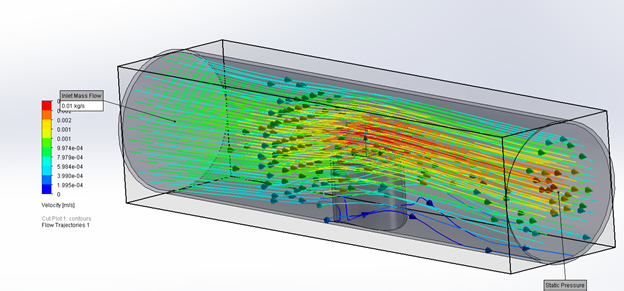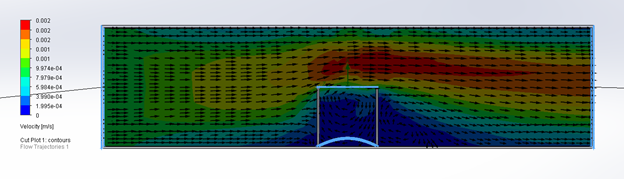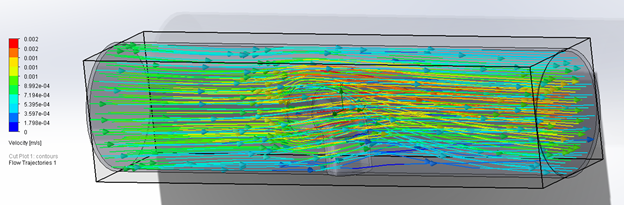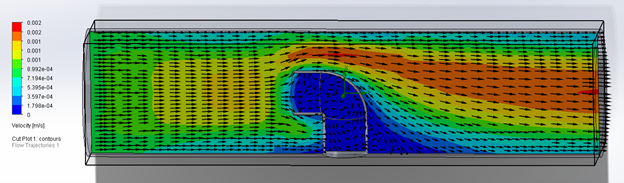# Different Pressures in SOLIDWORKS Flow Simulation

Article by Taran Packer on Dec 24, 2020

There are four different pressure definitions used in SOLIDWORKS Flow Simulation:

1. Static Pressure
2. Total Pressure
3. Dynamic Pressure
4. Environment Pressure

## Static Pressure

Static Pressure is the pressure at a single point in the moving flow field as the gas or liquid flow moves through a system. Static Pressure can be measured as the flow passes over a device that introduces no velocity change to the flow or a device moving with the flow field.

An example of this is a measuring device inside of an open tube parallel to the fluid flow, shown below, usually by a manometer or pressure gauge that is placed into the flow field via a small hole that is drilled into the side of the tube.## Total Pressure

Total Pressure is the pressure of the fluid flow that is not moving but is at rest. It is also called Stagnation Pressure. The measurement tube for Total Pressure is an impact tube that is pointing directly into the flow field as in the example below. The fluid can move into the tube but since there is no outlet, it stagnates and in this resting condition the pressure is measured to give the Total Pressure.

The Total Pressure can be mathematically obtained in fluid dynamics by summing the Static Pressure, Dynamic Pressure, and the Gravitational Head, as expressed by Bernoulli’s principle.

p0 p = q + ρgz

P is the density of the fluid, g is the local acceleration due to gravity, and z is the height of the stagnant flow field.## Dynamic Pressure

Dynamic pressure is equal to the difference between Static and Total Pressure. It is closely related to the kinetic energy of a single fluid particle in the flow field. It is one of the terms of Bernoulli’s equation, which is an equation of energy conservation for a flow field in motion. This pressure is not one of the boundary pressure input options, but it is a goal that can be tracked as an output.

## Environment Pressure

Environment Pressure is not a physical pressure that can be measured in the real world. It is a boundary condition option within the virtual Flow Simulation environment. Flow Simulation interprets this pressure as a Total Pressure for inlet flow and as a Static Pressure for outlet flow.

Because of this ability of Environment Pressure to track inlet and outlet pressures, it is possible to accurately calculate a vortex that crosses an opening when this boundary condition is applied to it making it more accurate than a Static Pressure condition when a vortex is seen across an opening.

Backspin is Important to Your Basketball Free Throw! A SOLIDWORKS Simulation Study

Tank Sloshing Using SOLIDWORKS Flow Simulation

Beating COVID-19 with a Snorkel

Manifold Optimization with SOLIDWORKS Flow Simulation

Negative Pressure Rooms in Hospitals